# Comparing Fractions With Like Denominators: Lesson for Kids

Lesson Transcript
Instructor: Kelley Lipke

Kelley has been teaching middle school for six years and has a master's degree in educational administration.

When we compare things in math, we may say that something is equal to, greater than or less than something else. In this lesson, you will learn how to compare fractions with like denominators. Updated: 05/26/2020

## Fractions in Math

A fraction is a number that shows the parts of a whole. Fractions also represent numbers that are less than 1.

This model represents the fraction 1/4: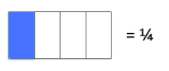Here, one out of a total of four squares is shaded.

Fractions are composed of two numbers: the numerator and denominator.

The numerator is the top number. It represents how many parts have been selected out of the total number of parts. In this case, 1 is the numerator because only one square has been shaded.

The denominator is the bottom number. It represents the whole, or total amount. Here, the number 4 is the denominator because the model is made up of a total of four squares.

Let's write a fraction for this model: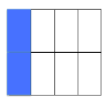Since two of the eight parts have been shaded, the fraction is 2/8 .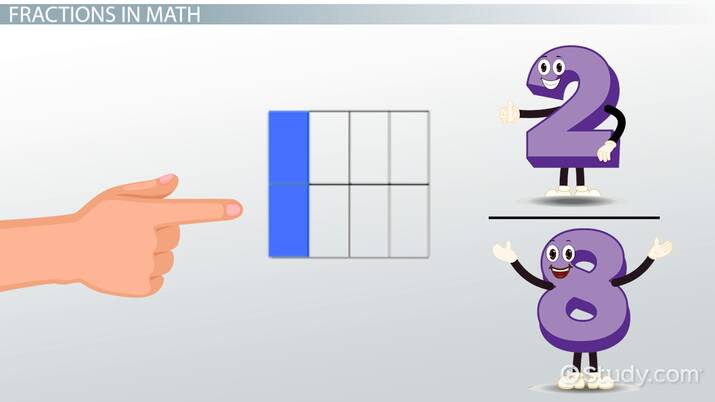An error occurred trying to load this video.

Try refreshing the page, or contact customer support.

Coming up next: How to Read & Write Decimals to the Hundredths Place: Lesson for Kids

### You're on a roll. Keep up the good work!

Replay
Your next lesson will play in 10 seconds
• 0:04 Fractions in Math
• 0:52 Like Denominators
• 1:44 Use of Symbols
• 2:12 Practice Problem
• 2:40 Lesson Summary
Save Save

Want to watch this again later?

Timeline
Autoplay
Autoplay
Speed Speed

## Like Denominators

Comparing fractions with like denominators is pretty easy. When we say like denominators, we are talking about fractions that have the exact same denominator, or bottom number.

For example, 3/8 and 6/8 have like denominators because they both have a denominator of 8.

Let's look at an example. Say your friend asks you if you want 3/5 of a pizza or 2/5 of a cake. You probably want the bigger piece, right?

But how do you know if there's more pizza or more cake? Since 3/5 and 2/5 have like denominators, they both have a total of five pieces. Now, all you have to do is look at the numerators, which will tell you how many pieces you'll get out of a total of five pieces.

As 3 is more than 2, you'd want 3/5 of a pizza, rather than 2/5 of a cake.

Let's take a look: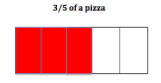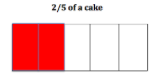## Use of Symbols

When comparing fractions, we usually use symbols to show if a fraction is less than, or greater than, or equal to another fraction.

To unlock this lesson you must be a Study.com Member.

### Register to view this lesson

Are you a student or a teacher?